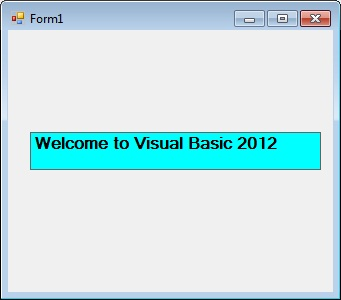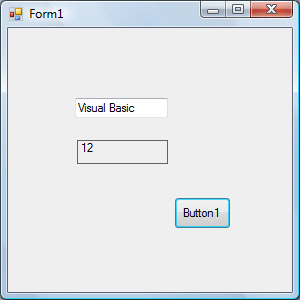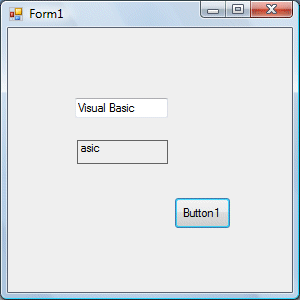# Visual Basic 2012 Lesson 8- String Manipulation

### 8.1 String Manipulation Using + and & signs.

In Visual Basic 2012, strings can be manipulated using the & sign and the + sign, both perform the string concatenation which means combining two or more smaller strings into larger strings. For example, we can join “Visual”,”Basic” and “2012” into “Visual Basic 2012” using “Visual”&”Basic” or “Visual “+”Basic”, as shown in the Examples below

#### Example 8.1(a)

```Public Class Form1

Private Sub Button1_Click(ByVal sender As System.Object, ByVal e As System.EventArgs) Handles Button1.Click
Dim text1, text2, text3, text4 As String
text1 = "Visual"
text2 = "Basic"
text3="2012"
text4 = text1 + text2+text3
MsgBox(text4)

End Sub
End Class
```

The line text4=text1+ text2 + text3 can be replaced by text4=text1 & text2 &text3 and produces the same output. However, if one of the variables is declared as numeric data type, you cannot use the + sign, you can only use the & sign.

#### Example 8.1(b)

```Dim text1, text3 as string
Dim Text2 As Integer

text1 = "Visual"
text2=22
text3=text1+text2
Label1.Text = text3
```

This code will produce an error because of data mismatch.However, using & instead of + will be all right.

```Dim text1, text3 as string
Dim Text2 As Integer
text1 = "Visual"
text2=22
text3=text1 & text2
Label1.Text = text3
```

You can combine more than two strings to form a larger string, like the following example:

```Public Class Form1

Private Sub Button1_Click(ByVal sender As System.Object, ByVal e As System.EventArgs) Handles Button1.Click
Dim text1, text2, text3, text4, text5, text6 As String
text1 = "Welcome"
text2 = " to"
text3 = " Visual"
text4 = " Basic"
text5 = " 2012"
text6 = text1 + text2 + text3+text4+text5
Label1.Text = text6
End Sub

End Class
```

Running the above program will produce the following screen shot.### 8.2 String Manipulation Using VB2012 Built-in Functions

A function is similar to a normal procedure but the main purpose of the function is to accept a certain input and return a value which is passed on to the main program to finish the execution.There are numerous string manipulation functions built into Visual Basic 2012 but I will only discuss a few here and will explain the rest of them in later lessons.

#### 8.2 (a) The Len Function

The Len function returns an integer value which is the length of a phrase or a sentence, including the empty spaces. The syntax is
Len (“Phrase”)

For example,

```Len (Visual Basic) = 12
```
```and
Len ("welcome to VB tutorial") = 22
```

Example 8.3

Public Class Form1

```Private Sub Button1_Click(ByVal sender As System.Object, ByVal e As System.EventArgs) Handles Button1.Click
Label1.Text = Len(TextBox1.Text)
End Sub
End Class
```

The output:#### 8.2(b) The Right Function

The Right function extracts the right portion of a phrase. The format for Visual Basic 6 is

```Right ("Phrase", n)
```

Where n is the starting position from the right of the phase where the portion of the phrase is going to be extracted. For example,

```Right("Visual Basic", 4) = asic
```

However, this syntax is not applicable in VB2012. In VB2012, we need use the following format

```Microsoft.VisualBasic.Right("Phrase",n)
```

#### Example 8.2(a)

```Private Sub Button1_Click(ByVal sender As System.Object, ByVal e As System.EventArgs) Handles Button1.Click
Dim text1 As String
text1 = TextBox1.Text
Label1.Text = Microsoft.VisualBasic.Right(text1, 4)
End Sub
```

The above program returns four rightmost characters of the phrase entered into the textbox.

The Output:*The reason of using the full reference is because many objects have the Right properties so using Right on its own will make it ambiguous to Visual Basic 2012.

#### 8.2(c)The Left Function

The Left function extracts the left portion of a phrase. The syntax  is

`Microsoft.VisualBasic.Left("Phrase",n)`

Where n is the starting position from the left of the phase where the portion of the phrase is going to be extracted. For example,

Microsoft.VisualBasic.Left (“Visual Basic”, 4) = Visu .